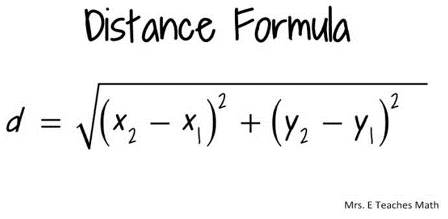Back to Tracking Project - Intro

Back to Research Questions

## To measure distance:

1. If you have the GPS coordinates of two locations in UTMs, you can use the following formula to calculate the distance between the two points (x1, y1) and (x2, y2):2. In Google Earth, use the measuring icon on the toolbar (shown below), or go to the Tools menu and click on Ruler.## To calculate area:

To calculate the area of a polygon in Google Earth, and you do not have Google Earth Pro, you can use the following website: http://www.earthpoint.us/Shapes.aspx

## Physics:

Velocity= meters/second, miles/hour Acceleration is change in speed over time; (meters/second)/second; meters/second2 Force = mass X acceleration Watts=Joules/second

More about force, work, energy, and power concepts can be found here: http://hyperphysics.phy-astr.gsu.edu/hbase/work.html